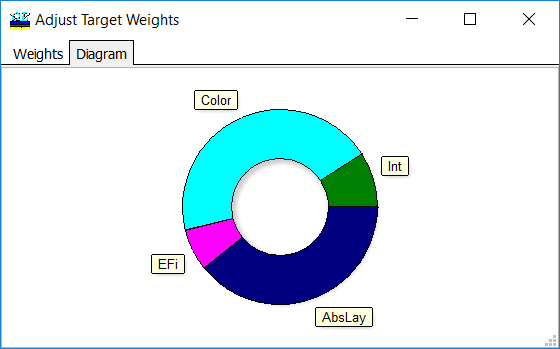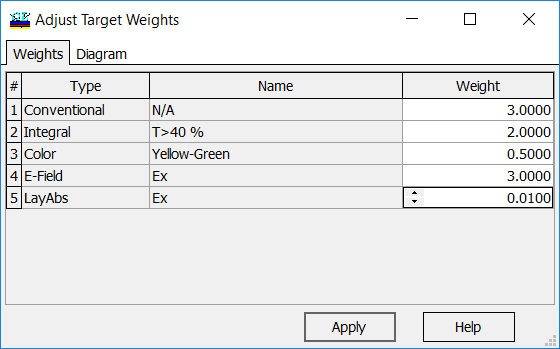### Combined Targets

 OptiLayer allows combining targets of different types, namely conventional targets (also range targets), integral targets, color target, electric field target and layer absorbtance target. Resulting Merit Function value is computed with formula: $MF^2=w_1 MF_{conv}^2+w_2 MF_{col}^2+w_3 MF_{int}^2+$ $+w_4 MF_{EFI}^2+w_5 MF_{LA}^2+w_6 MF_{Th/Stress},$ where $$w_1, w_2, w_3, w_4$$ and $$w_5$$ are target weights. Adjust Target Weights dialog allows you to change relative weight of every target loaded to memory. It is convenient and simple way to adjust the importance of different requirements in complicated problems. In the diagram tab the contribution of each term is displayed. Adjusting weights it is possible to control the relative importance of different target requirements in multi-criteria design problems.